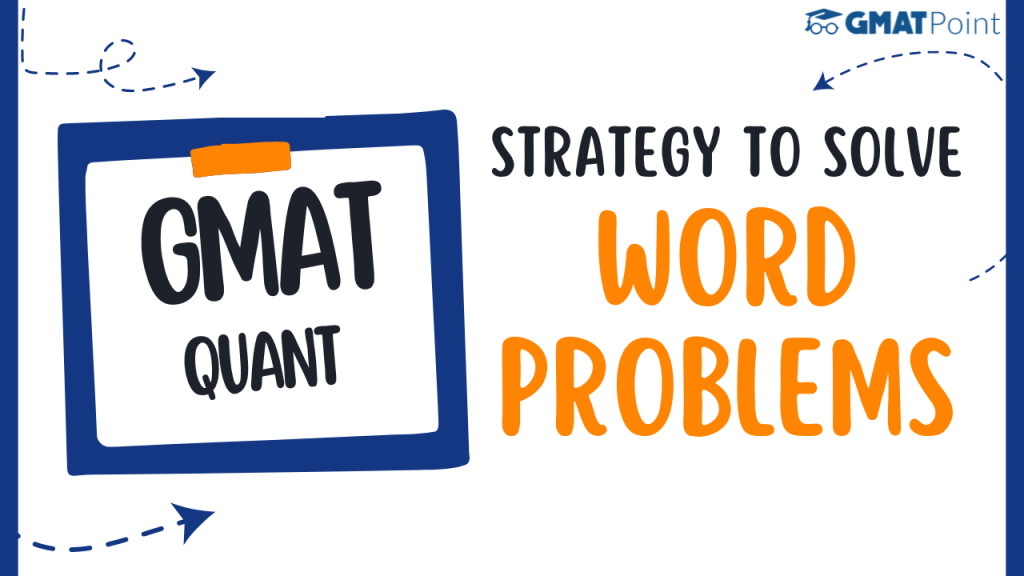# Strategy For Solving Word Problems In GMAT QuantStudents with strong language abilities tend to perform well on the GMAT for a significant reason – the Quantitative section of the exam heavily relies on English comprehension. The GMAT’s Quantitative section consists primarily of Word Problems, where symbolic representations are minimal, and language plays a substantial role in both the Problem-Solving and Data Sufficiency formats.

This aspect poses a challenge for non-native English speakers who take the test, as they often need help integrating their English language skills with mathematical and verbal abilities. For instance, Asian test-takers, especially those from India, typically approach Math problems using notational or algebraic language rather than English.

To achieve a high score of 50 or more on the Quantitative section, test-takers must master the skill of converting English into logic or algebra. This post will address the necessary steps to develop this skill and solve seemingly lengthy problems efficiently.

## Resist the urge to directly convert words into equations as you read from left to right

Let’s examine the given statement:

“There are five times as many bulls as there are cows.”

Test-takers who are accustomed to immediately converting sentences into equations might be tempted to represent this as 5B = C since the terms “five,” “bulls,” and “cows” appear from left to right in that order. However, the first step is to avoid this instinct and instead focus on understanding the logic of the statement.

By analyzing the statement, we can determine that there are more bulls than cows. Therefore, the correct representation of the statement should be B = 5C. Only after grasping the logic should you frame an equation, if necessary.

## Don’t Start Framing Equations Till You Reach The End of the Problem

An important lesson for test-takers is to refrain from immediately framing equations while reading a word problem. Many people tend to convert each sentence into an equation as they read through the problem, but this approach can be detrimental, especially in the Math section of an aptitude test.

A common mistake is assuming that the information is presented in a linear format and building a solving structure based on this assumption. In reality, questions, particularly the moderate to tougher ones, may not present information in a sequential manner.

For instance, after providing four lines of information, the question might suddenly be asked, “In how many days will the job be completed if Amit leaves after the sixth day?”

Jumping into solving the problem prematurely is a pitfall to avoid. Doing so means creating a solving structure without knowing the full context of the problem, including what is being asked and any potential twists that might be revealed later.

This premature approach can lead to two main issues:

1. Creating a more complex equation than necessary.
2. Creating an equation with the wrong variables.

To prevent such mistakes, lesson number two is to wait to frame the equations until you’ve read the entire problem and understood its complete context. This way, you can approach the problem with a clear understanding of what must be solved and avoid unnecessary complications or errors in your equations.

## Write equations using the variable you need to determine, not the first variable you encounter

When solving problems, using the variable you need to determine is crucial, not the first variable you encounter. Reading the entire problem before starting to write equations helps one understand the task at hand and anticipate any hidden twists.

For instance, let’s consider the problem of Anushka’s take-home pay and savings. Many test-takers may rush to write equations using variables like X for salary and Y for savings based on the statement: “The total amount she saved at the end of the year was 3 times the amount of that portion of her monthly take-home that she did not save.”

However, the problem is asking for a fraction of her take-home pay that she saved each month (let’s call it F). It makes more sense to write the equation using F and her salary (S): 12FS = 3(1-F)S or F = 1/5.

By using the variable you need to determine (F), you avoid falling into traps set by answer options corresponding to other variables. Always take what the question asks for (e.g., F in this case) and express everything else in terms of that variable.

Another approach is to directly process the words into logic, avoiding the use of unnecessary variables. For example, the statement “The total amount she saved at the end of the year was 3 times the amount of that portion of her monthly take-home that she did not save” can be translated into “Twelve times her monthly savings is equal to three times her monthly expenditure.”

This logic reveals that her monthly expenditure is four times her monthly savings, indicating her monthly salary is five times her monthly savings. This simplifies the problem and helps find the answer efficiently.